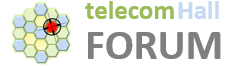# Why 100 PRB in LTE 20 MHz?

Hi All,

According to 3GPP specifications LTE channel bandwidth can be 1.4, 3, 5, 10, 15, 20 MHz.

All available spectrum is divided into Resource Blocks (RB).

For example, for Channel Bandwidth = 20 MHz we have 100 Resources Blocks.

My question is: where does this 100 PRB comes from (what is the calculation)?

Because we have:

1 PRB (in frequency domain) = 12 subcarriers
1 subcarrier = 15 KHz
12 subcarriers = 180 KHz
Bandwidth = 20 MHz = 20,000 KHz
20,000 / 180 = 111.111

Anyone can shed a light?

2 Likes

10% is a guard band, it is not 20MHz, actually it is 18MHz

2 Likes

Those 100PRBs are used for PDSCH channels only. You forgot to include the other channels and guard bands

2 Likes

Because of the Guard Band (narrow frequency range that separates two ranges of wider frequency, in order to avoid interference which would result in decreased quality for transmission).

In LTE Guard Band is 10% of available bandwidth.

So, in the 20 MHz: 10% = 2.000 KHz.

And remaining is 18.000 KHz.

So: 18.000 / 180 = 100

Note: it do not apply for 1.4 MHz

3 Likes

Relation between Bandwidth and Resource Block:

Bandwidth directly affects the throughput. Different BWs have different number of RBs.Here is the calculation how to find out the numbers of subcarriers and Resource Blocks.10% of total bandwidth is assumed to be used for guard band. Though 10 % guard band assumption is not valid for 1.4 MHz bandwidth. Let’s take an example of 20MHz.10% of 20 MHz is 2 MHz, used as guard band, thus effective bandwidth will be 18MHz. Number of subcarriers = 18 MHz/15KHz = 1200. Number of Resource Blocks =18 MHz/180KHz = 100. Same calculations can be done with other bandwidths to calculate the number of subcarriers and Resource Blocks.

1 Like